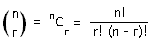## Permutations and Combinations

This section covers permutations and combinations.

Arranging Objects

The number of ways of arranging n unlike objects in a line is n! (pronounced ‘n factorial’). n! = n × (n – 1) × (n – 2) ×…× 3 × 2 × 1

Example

How many different ways can the letters P, Q, R, S be arranged?

The answer is 4! = 24.

This is because there are four spaces to be filled: _, _, _, _

The first space can be filled by any one of the four letters. The second space can be filled by any of the remaining 3 letters. The third space can be filled by any of the 2 remaining letters and the final space must be filled by the one remaining letter. The total number of possible arrangements is therefore 4 × 3 × 2 × 1 = 4!

• The number of ways of arranging n objects, of which p of one type are alike, q of a second type are alike, r of a third type are alike, etc is:

n!        .
p! q! r! …

Example

In how many ways can the letters in the word: STATISTICS be arranged?

There are 3 S’s, 2 I’s and 3 T’s in this word, therefore, the number of ways of arranging the letters are:

10!=50 400
3! 2! 3!

• The number of ways of arranging n unlike objects in a ring when clockwise and anticlockwise arrangements are different is (n – 1)!

When clockwise and anti-clockwise arrangements are the same, the number of ways is ½ (n – 1)!

Example

Ten people go to a party. How many different ways can they be seated?

Anti-clockwise and clockwise arrangements are the same. Therefore, the total number of ways is ½ (10-1)! = 181 440

Combinations

The number of ways of selecting r objects from n unlike objects is:Example

There are 10 balls in a bag numbered from 1 to 10. Three balls are selected at random. How many different ways are there of selecting the three balls?

10C3 =10!=10 × 9 × 8= 120
3! (10 – 3)!3 × 2 × 1

Permutations

A permutation is an ordered arrangement.

• The number of ordered arrangements of r objects taken from n unlike objects is:

nPr =       n!       .
(n – r)!

Example

In the Match of the Day’s goal of the month competition, you had to pick the top 3 goals out of 10. Since the order is important, it is the permutation formula which we use.

10P3 =10!
7!

= 720

There are therefore 720 different ways of picking the top three goals.

Probability

The above facts can be used to help solve problems in probability.

Example

In the National Lottery, 6 numbers are chosen from 49. You win if the 6 balls you pick match the six balls selected by the machine. What is the probability of winning the National Lottery?

The number of ways of choosing 6 numbers from 49 is 49C6 = 13 983 816 .

Therefore the probability of winning the lottery is 1/13983816 = 0.000 000 071 5 (3sf), which is about a 1 in 14 million chance.